# Semi-continuous mapping

(diff) ← Older revision | Latest revision (diff) | Newer revision → (diff)

upper (lower)

A mappingfrom a topological spaceinto a partially ordered setsuch that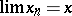implies that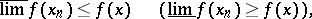where() denotes the limes superior (inferior).

On a partially ordered setthe collection consisting ofand all sets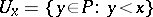is a base for a topology on, denoted by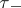, andand all setsdefine a topology. The mapping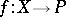is upper semi-continuous, (u.s.c.) (respectively, lower semi-continuous (l.s.c.)) if and only if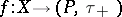(respectively,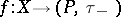) is continuous.
In fact, upper and lower semi-continuity are usually defined only for mappings to the real line. In terms of open sets, one sees thatis upper (lower) semi-continuous if and only if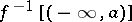() is open for every.
Semi-continuity is also defined for set-valued mappings. A mapping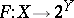is upper (lower) semi-continuous if for every open subsetofthe set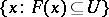(the set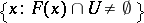) is open.
Note that if a mappingis regarded as a set-valued mapping,, thenis lower semi-continuous if and only ifis lower semi-continuous; andis upper semi-continuous if and only ifis upper semi-continuous.